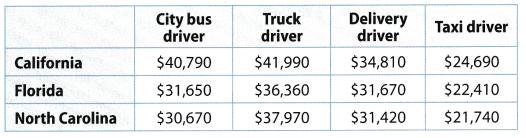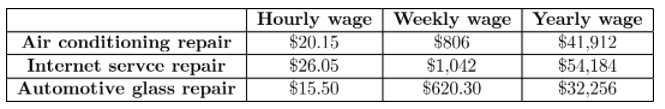# Texas Go Math Grade 6 Lesson 18.4 Answer Key Wages, Salaries, and Careers

Refer to our Texas Go Math Grade 6 Answer Key Pdf to score good marks in the exams. Test yourself by practicing the problems from Texas Go Math Grade 6 Lesson 18.4 Answer Key Wages, Salaries, and Careers.

## Texas Go Math Grade 6 Lesson 18.4 Answer Key Wages, Salaries, and Careers

Explore Activity 1

Exploring Salaries and Careers

The U.S. Bureau of Labor Statistics website can help you research the salary and educational requirements for a variety of occupations. Look up each of these occupations and complete the table.Reflect

Question 1.
Besides salary, what are some other things you might consider in choosing a career?
Some things that might be considered in choosing a career are the following: nature of work, location, working hours, and benefits.

Explore Activity 2

Choosing a Career

Use the Bureau of Labor Statistics website to research the career you are considering. Read about its median income, educational requirements, and job outlook. Then complete the bubble map.Example 1

The annual median income for an economist is $89,450. The annual median income for a software developer is$90,530. Compare the salaries of these careers over 30 years. How much more can a software developer expect to earn over that time?STEP 1: Estimate the total income of an economist over 30 years.
$89,450 × 30 =$2,683,500 Multiply the annual income by 30.

STEP 2: Estimate the total income of a software developer over 30 years.
$90,530 × 30 =$2,715,900 Multiply the annual : income by 30.

STEP 3: Find the difference between these total incomes.
$2,715,900 –$2,683,500 = $32,400 A software developer can expect to earn about$32,400 more than 6 an economist over 30 years.

Reflect

Question 2.
Communicate Mathematical Ideas What is another way to find how much more the software developer can earn over 30 years?
Identify another way to compare the income over 30 years.
$90,530 –$89,450 = $1,080 subtract the income of developer from the economist$1,080 × 30 = $32, 400 difference of income over 30 years Another way of comparing their income is to subtract both income of the developer and economist then multiply by the number of years. Question 3. Select Tools What are two tools you could use to find the total income over 30 years? Answer: The operation used from the previous calculation is subtraction and multiplication. Subtract both incomes then multiply the difference by the number of years. Your Turn Question 4. The annual median income for a dental assistant is$33,470. The annual median income for a nursing aide is $25,010. How much more can a dental assistant expect to earn than a nursing aide over 30 years? Answer: Compare the income over 30 years.$33,470 $25,010 =$8,460 subtract the income of nursing aide from the dental assistant
$8,460 × 30 =$253,800 difference of income over 30 years
A dental assistant will earn $253,800 more than the nursing aide over 30 years Texas Go Math Grade 6 Lesson 18.4 Guided Practice Answer Key The table shows information about two occupations.Question 1. Compare and contrast these two occupations based on the information in the table. (Explore Activities 1 and 2) Answer: Both occupations requires for a degree holder employee. However, a construction manager has an educational requirement of an associate degree while a microbiologists must hold a bachelor degree. The median income of a construction manager is higher than the microbiologist. A microbiologist needed a specific area to fulfill his work while a construction manager may work from a field office of a construction site or at home where he can do paperworks. Question 2. Using the information from the table, determine how much less a microbiologist would earn over 30 years than a construction manager. (Example 1) Answer: Compare the income over 30 years.$83,860 – $65. 920 =$17,910 subtract the income of microbiologists from the construction manager.
$17, 940 × 30 =$538, 200 difference of income over 30 years
A microbiologist will earn $538,200 less than the construction manager over 30 years. Essential Question Check-In Question 3. How can you compare salaries of different occupations? Answer: By looking at the figures, salaries can easily be compared by the amount indicated for each occupation. If it is over a certain period of time, subtract the income for both occupations then multiply it by the given period of time. Texas Go Math Grade 6 Lesson 18.4 Independent Practice Answer Key Question 4. Simon earns$2,470 per month and Amaress earns $2,340 per month. Amaress also gets a yearly bonus in the amount of$750.
a. How much does Simon earn per year?
Determine the amount Simon earns per year.
$2,470 × 12 =$29,640 amount per month multiplied by 12
Simon earns $29,640 in a year. b. How much does Amaress earn per year? Answer: Determine the amount Amress earns per year$2,340 × 12 = $28,080 amount per month multiplied by 12$28,080 + $750 =$28,830 amount Amress earns per year
Amaress earns $28,830 in a year. c. What is the difference between the amount that Simon could earn in 30 years and the amount that Amaress could earn in 30 years? Answer: Compare their earnings in 30 years.$29,640 × 30 = $889,200 amount that Simon earns in 30 years$28,830 × 30 = $864,900 amount that Amaress earns in 30 years$889,200 – $864,900 =$24,300 difference in their earnings in 30 years
Simon earns $24,300 more than Amaress in 30 years. For 5-7, use the table of median yearly income for various kinds of drivers in three states that Timothy is interested in moving to.Question 5. How much can Timothy earn in 30 years as a delivery driver in California? Answer: Determine the amount that Timothy earns as a delivery driver.$34,810 × 30 = $1,044,300 earnings in 30 years as a delivery driver Timothy will, earn$1,044,300 in 30 years as a delivery driver.

Question 6.
Compare the salaries of a Florida truck driver and a North Carolina city bus driver. How much more money can Timothy earn as a Florida truck driver in 30 years?
Compare the earnings of a Florida truck driver with a North Carolina city bus driver in 30 years.
$36,360 × 30 =$1,090,800 earnings in 30 years as a truck driver
$30,670 × 30 =$920,100 earnings in 30 years as a city bus driver
$1,090. 800 –$920,100 = $170,700 difference on the earnings Timothy will earn$170,700 more as a Florida truck driver than a North Carolina city bus driver in 30 years.

Question 7.
What is the difference between the income earned over 30 years at the highest paying job and the income earned over 30 years at the lowest paying job?
Compare the earnings of the highest paying job and lowest paying job over 30 years.
$41,990 × 30 =$1,259,700 earnings in 30 years of a California truck driver
$21,740 × 30 =$652,200 earnings in 30 years of a North Carolina taxi driver
$1, 259,700 –$652,200 = $607,500 difference on the earnings The difference between the highest and lowest paying job over 30 years is$607,500.

Question 8.
Harris and Georgina both work in a clothing store. Harris earns $2,360 per month and Georgina earns$2,120 per month. Every month, the employee with the highest sales gets a $250 bonus. In the past year, Georgina got the monthly bonus 7 times, and Harris got the monthly bonus 1 time. a. How much did Harris earn in the past year? Explain. Answer: Determine the amount Harris earns in the past year.$2,360 × 12 = $28,320 earnings in a year$28,320 – $250 =$28,570 total earnings with one month bonus
Harris earns $28,570 with 1 month bonus in the past year. b. How much did Georgina earn in the past year? Explain. Answer: Determine the amount Georgina earns in the past year.$2,120 × 12 = $25,440 earnings in a year$250 × 7 = $1,750 monthly bonus$25,440 + $1,750 =$27,190 total earnings with 7 months bonus
Georgina earns $27,190 with 7 months bonus in the past year. Question 9. Janelle earns$950 a week. Carter earns $50,320 a year. Each works 52 weeks a year. What is the difference between the amounts Janelle and Carter earn in 30 years? Explain. Answer: Compare their earnings ¡n 30 years.$950 × 52 = $49,400 earnings of Janelle in a year$49, 400 × 30 = $1,482,000 earnings of Janelle in 30 years$50, 320 × 30 = $1,509, 600 earnings of Carter in 30 years$1, 509,600 – $1,482,000 =$27,600 difference between the earnings of Janelle and Carter
Carter earns $27,600 more than Janelle in 30 years. Rayshawn is trying to decide between three job offers. Each job offer is 40 hours a week and 52 weeks a year. The table shows the wage for each of Rayshawn’s three job offers.Question 10. Calculate the hourly, weekly, and yearly wages to complete the table. Answer:Complete the table by calculating the hourly, weekly, and yearly wages. Question 11. What is the difference between the yearly wage of the internet service repair offer and the yearly wage of the air conditioning repair offers? Answer: Compare the yearly wage of internet service repair and air conditioning repair.$54,184 – $41,912 =$12,272 difference between the yearly wage
The yearly wage of internet service repair is greater by $12,272 than the air conditioning repair. H.O.T. Focus on Higher Order Thinking Question 12. Critique Reasoning Carlotta earns$2,400 a month. She thinks she will earn $720,000 in 30 years. Is Carlotta correct? Explain. Answer: Determine if Carlotta is correct in her conjecture.$2,400 × 12 = $28,800 earnings of Carlotta in a year$28,800 × 30 = $864,000 earnings of Carlotta in 30 years Carlotta is not correct because her earnings in 30 years is$864,000.

Question 13.
Communicate Mathematical Ideas If you know a job pays k dollars per hour, how can you use unit rates to find the job’s yearly salary? Explain.